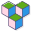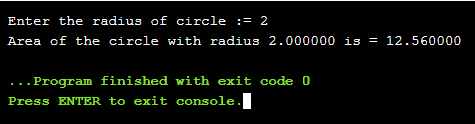CppBuzz# C program to find area of a Circle

The formula to calculate area of Circle is pi * radius * radius, where the value is pi is 3.14. In this program user is asked to enter radius and the output is the area of circle.

``````
#include<stdio.h>

#define PI 3.14

int main ()
{

printf("Enter the radius of circle := ");